﻿

#### Abstract

For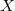$X$ a Hausdorff zero-dimensional topological space and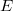$E$ a Hausdorff  non-Archimedean locally convex space, let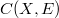$C(X,E)$ (resp.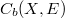$C_{b}(X,E)$) be  the space of all continuous (resp.  bounded continuous )$E$-valued functions  on$X$. Some of the properties of the spaces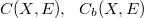$C(X,E),\ \ C_{b}(X,E)$,  equipped with certain locally convex topologies, are studied. Also, some  complete spaces of measures, on the algebra of all clopen subsets of$X$, are  investigated.

DOI Code: 10.1285/i15900932v30n1p61

Keywords:
Non-Archimedean fields; zero-dimensional spaces; Banaschewski compactification; locally convex spaces

Classification: 46S10; 46G10

Full Text: PDF